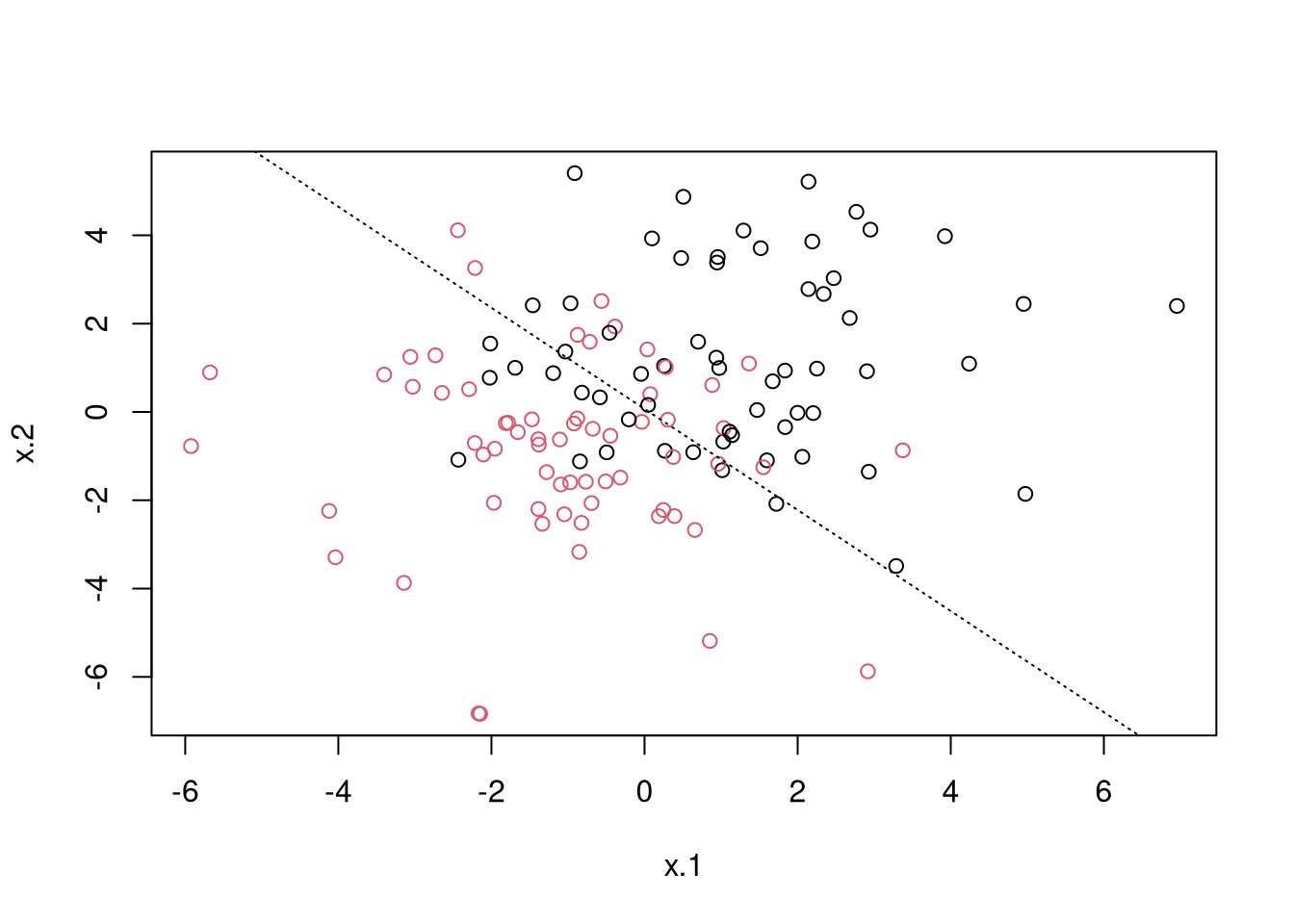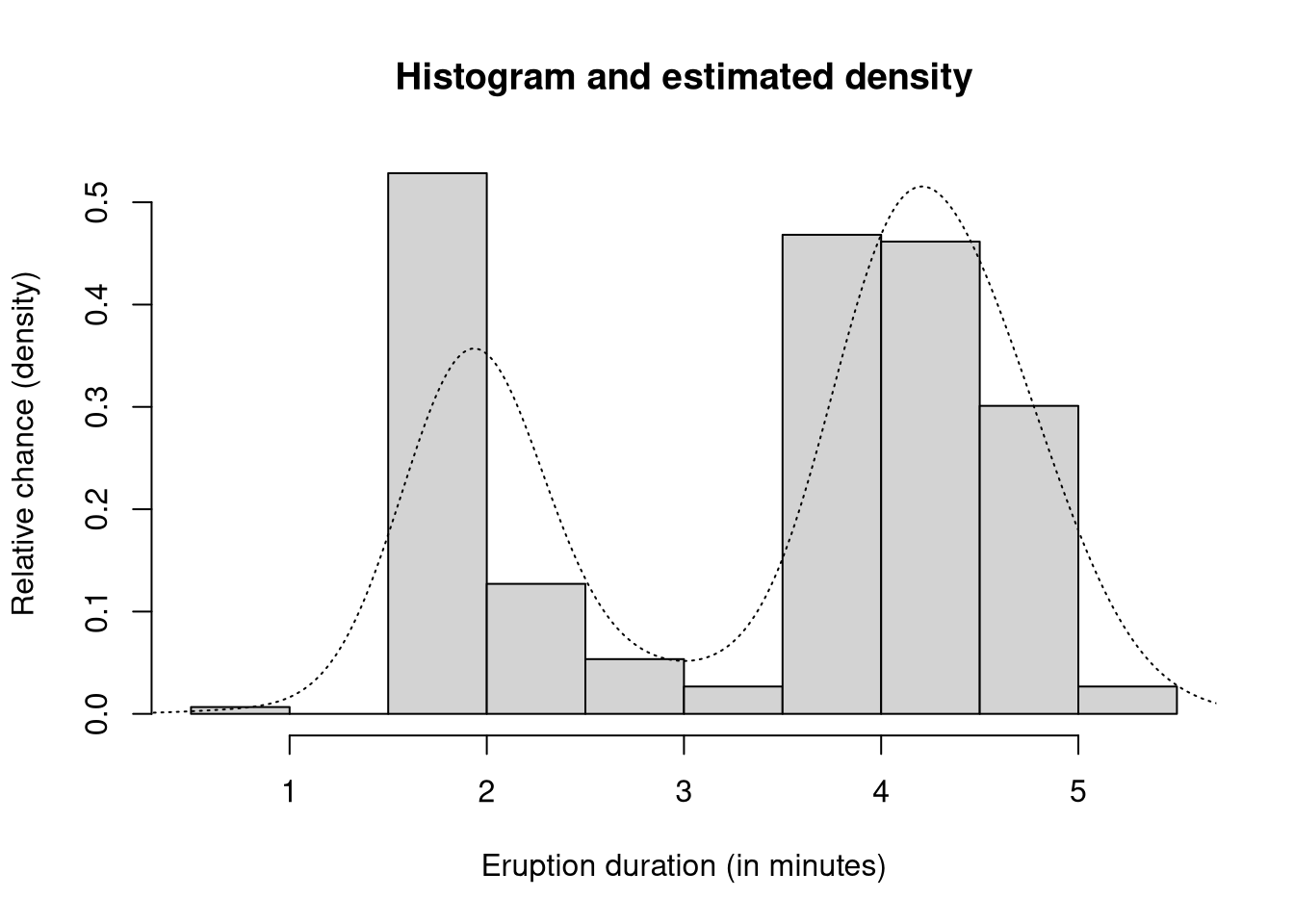# 计算机代写｜STAT5003 Computer Test – Example responses

### Question 1

Construct and analyze a binary classifier from a simulated dataset using your SID and the `mlbench` package.

1. [4 marks] Simulate a single dataset of `n = 500` observations completing the code below replacing `<insert SID here>` with your SID respectively and changing the `eval = FALSE` setting to `eval = TRUE` in the `R` code chunk. Then, inspect the `q2.dat` using the `head` command and verify the dimension of the `data.frame` and that there are 2 numeric features and a single factor variable of class labels. NoteYou should explicitly verify the data type is numeric or factor or inspect the class of each column.
``````library(mlbench)
set.seed(5003)
simulated.data <- mlbench.2dnormals(n = 500, sd = 2)
q2.dat <- as.data.frame(simulated.data)
``````##          x.1        x.2 classes
## 1  2.3110637 -2.4235455       1
## 2 -2.1471013 -6.8373623       2
## 3 -3.4813172 -1.2873004       2
## 4 -1.4097769  2.1890729       2
## 5  0.1110965  0.7407209       1
## 6  0.1862293  2.3522997       1``````
``dim(q2.dat)``
``##  500   3``
``````# Any of the below are acceptable
lapply(q2.dat, class)``````
``````## \$x.1
##  "numeric"
##
## \$x.2
##  "numeric"
##
## \$classes
##  "factor"``````
``str(q2.dat)``
``````## 'data.frame':    500 obs. of  3 variables:
##  \$ x.1    : num  2.311 -2.147 -3.481 -1.41 0.111 ...
##  \$ x.2    : num  -2.424 -6.837 -1.287 2.189 0.741 ...
##  \$ classes: Factor w/ 2 levels "1","2": 1 2 2 2 1 1 1 1 2 1 ...``````
1. [6 marks] Split the simulated data into 75% training and 25% test data set using `caret::createDataPartition` or otherwise. Then fit a logistic regression model to explain the classes response using the two features on the training data. Predict the classes on the test data using your fitted logistic regression model and the threshold for classification using the esimated probability of being in the positive class at 0.5. Compute the accuracy on the test set in this situation.
``````train.ind <- caret::createDataPartition(q2.dat[["classes"]], p = 0.75)[]
training.data <- q2.dat[train.ind, ]
test.data <- q2.dat[-train.ind, ]
trained.model <- glm(classes ~ ., data = training.data, family = binomial(link = "logit"))
predicted.classes <- ifelse(predict(trained.model, newdata = test.data) > 0.5, 2, 1)
accuracy <- mean(predicted.classes == test.data[["classes"]])
accuracy``````
``##  0.8225806``
1. Create a scatter plot of the data in the test set and colour each point by its true class label. Draw the linear decision boundary generated by the logistic regression model fitted above. Comment on how the accuracy computed on the test set in part c. relates to your plot and decision boundary.
``````plot(x.2 ~ x.1, data = test.data, col = classes)
betas <- coef(trained.model)
abline(a = -betas/betas, b = -betas/betas, lty = "dotted")``````The model will classify points in the bottom left of the plot (below the boundary) as being in the positive class and points in the top right (above the boundary) as the negative class. These classifications are correct 82.2580645% of the time.

### Question 2

Consider the estimation of density of the duration of a geyser eruptions (in minutes). Provided is a messy and clean dataset these geyser eruption durations in the files `clean-s1-22-q1.rds` and `messy-s1-22-q2.rds` respectively. The `readRDS` commands below load the data using the native data format in `R`.

``````messy.duration <- readRDS('messy-s1-22-q2.rds')

Suppose only the messy dataset was only available initially and requires cleaning by removing the negative and missing (`NA`) values. The goal here is the clean the messy dataset and then provide an analysis of the stability of the bandwidths in the `density` algorithm.

1. [4 marks] Some geyser eruptions were recorded incorrectly with a negative duration or were coded as missing (coded as `NA`). Using relevant `R` code, verify that `messy.duration` is a `numeric` vector with `341` observations. Also count the number of observations that are negative and the number that are coded as missing (i.e. `NA`).
``length(messy.duration)``
``##  341``
``````n.missing <- sum(is.na(messy.duration))
n.negative <- sum(messy.duration < 0, na.rm = TRUE)
c(`n missing` = n.missing, `n negative` = n.negative)``````
``````##  n missing n negative
##         22         20``````
1. [ marks] Create a new vector called `my.cleaned.duration` which uses the `messy.duration` vector and cleans it by removing the negative values or values that are coded as `NA`Verify that your created vector is the same as the `clean.duration` dataset using a call to `all.equal` or `identical`.
``````my.cleaned.duration <- messy.duration[!is.na(messy.duration) & messy.duration > 0]
identical(my.cleaned.duration, clean.duration)``````
``##  TRUE``

From this point onwards, answer the questions using only the `clean.duration` dataset.

1. [6 marks] Produce a histogram and kernel density estimate of the cleaned data on the same plot. Using either the base `R` plotting commands (e.g. `hist`) or using the `ggplot2` comments are fine, no need to use both. Also no need to do bandwidth selection in this question and any default bandwidths are fine.
``````hist(clean.duration, xlab = "Eruption duration (in minutes)", ylab = "Relative chance (density)",
prob = TRUE, main = "Histogram and estimated density")
lines(density(clean.duration), col = 1, lty = "dotted")``````1. [2 marks] Construct `B = 341` bootstrap samples of the cleaned geyser data by resampling with replacement (i.e. using the functions `sample` or `sample.int`, marks will not be awarded for code that uses an external package like `boot`).
``````set.seed(5003)
B <- 341L
n.valid <- 299L
bootstrapped.indices <- replicate(B, sample.int(n.valid, replace = TRUE, size = n.valid), simplify = FALSE)
bootstrapped.geyser <- lapply(bootstrapped.indices, \(x) clean.duration[x])``````
1. [3 marks] Using your bootstrapped geyser data above, analyze how variable the default bandwidth estimator is on this bootstrapped data. Visualize your results using a boxplot (again using either the base `boxplot` command or `ggplot2` suite are fine, no need to do both). Hint: This can be done by extracting the `bw` element of the return output of `density` or by calling the function `bw.nrd0` instead.
``````default.bandwidths <- vapply(bootstrapped.geyser, bw.nrd0, numeric(1L))
boxplot(default.bandwidths, main = "Bootstrapped default bandwidth selection on the Geyser data")``````E-mail: itcsdx@outlook.com  微信:itcsdx